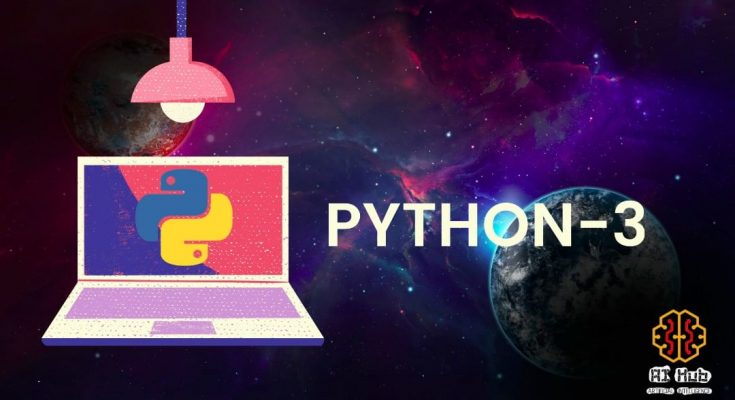# PYTHON PROGRAMMING EXAMPLES##### WRITE A PROGRAM TO CHECK PRIME NUMBERS
`````` >>> a = int (input("enter a number : "))
>>> c = 0
>>> if (a<1):
print("invalid input ! Try next number ")
>>> if(a==1):
print ("1 is not prime")
>>> else:
for i in range(2,a,1):
if (a%i==0):
c=1
if (c==0):
print(a," is prime")
else:
print(a," is not prime")

Output:
enter a number : 9
9  is not prime``````
##### WRITE A PROGRAM TO FIND FIBONACCI SERIES
``````# print fibonacci series
>>> a = 1
>>> b = 0
>>> n = int(input("how many fibonacci series ?? "))
>>> while (n>=1):
c = a+b
a = b
b = c
print (c, end=",")
n=n-1

Output:
how many fibonacci series ?? 5
1,1,2,3,5``````

``````# print nth fibonacci series value
>>> a = 1
>>> b = 0
>>> n = int(input("how many fibonacci series ?? "))
>>> while (n>=1):
c = a+b
a = b
b = c
n=n-1
>>> print (c,"is the required output")

Output:
how many fibonacci series ?? 6
8 is the required output``````
##### WRITE A PROGRAM TO CHECK ARMSTRONG NUMBER
`````` >>> #153 is an Armstrong number.
>>> #1*1*1 + 5*5*5 + 3*3*3 = 153
>>> sum = 0
>>> c = 0
>>> num = int(input("enter a number : "))
>>> temp = num
>>> while(num>0):               # to find length of number i.e. 153= 3
a = num%10
num= num//10
c=c+1
>>> num = temp
>>> while(num>0):              # 1*1*1+5*5*5+3*3*3 = 153
a = num%10
num= (num-a)/10
sum = int(sum + a**c)
>>> if (sum == temp):
print("Armstrong Number")
>>> else:
print (" Not an armstrong number")

Output:
enter a number : 153
Armstrong Number``````

##### WRITE A PROGRAM TO FIND HCF OF TWO NUMBER
`````` >>> num1 = int (input ("enter first number : "))
>>> num2 = int (input ("enter second number : "))
>>> if num1 < num2:
small = num1
>>> else:
small = num2
>>> for i in range(1, small+1):
if((num1 % i == 0) and (num2 % i == 0)):
hcf = i
>>> print(" HCF or GCD of ",num1,"&", num2,"is",hcf)

Output:
enter first number : 3
enter second number : 5
HCF or GCD of  3 & 5 is 1``````
##### WRITE A PROGRAM TO FIND LCM OF TWO NUMBER
``````>>> num1 = int (input ("enter first number : "))
>>> num2 = int (input ("enter second number : "))
>>> if num1 < num2:
small = num1
>>> else:
small = num2
>>> for i in range(1, small+1):
if((num1 % i == 0) and (num2 % i == 0)):
hcf = i
>>> lcm = int ((num1*num2)/hcf)
>>> print(" LCM of ",num1,"&", num2,"is",lcm)

Output:
enter first number : 4
enter second number : 8
LCM of  4 & 8 is 8``````

##### WRITE A PROGRAM TO FIND FACTOR OF A NUMBER
``````# 25= 5*5
>>> a = int(input("enter a number : "))
>>> for i in range(2,a):
for j in range (2,a+1):
if (a%j==0):
print (j,end=",")
break
a = int(a/j)

Output:
enter a number : 4
2,2``````
##### WRITE A NUMBER TO FIND FACTORIAL OF A NUMBER
``````>>> # 5! = 5*4*3*2*1
>>> a = int(input("enter a number : "))
>>> c = 1
>>> if (a==0):
print (" factorial of 0 is 1 ")
>>> else:
for i in range(1,a+1):
c=c*i
>>> print ("\n factorial of ",a,"is",c)

Output:
enter a number : 4
factorial of  4 is 24``````
##### WRITE A PROGRAM TO PRINT ALL VOWELS OF STRING
``````>>> a = str(input("enter a string ")).lower()
>>> b = len(a)
>>> c = 0
>>> while (c<=b-1):
if a[c]=='a' or a[c]=='e' or a[c]=='i' or a[c]=='o' or a[c]=='u' :
print(a[c])
c=c+1

Output:
enter a string Diwas
i
a``````

##### WRITE A PROGRAM TO CHECK PALINDROME STRING
``````>>> a = str(input("enter a string : "))
>>> b = (a[::-1])
>>> if (a==b):
print("Palindrome number")
>>> else:
print(" Not Palindrome number")

Output:
enter a string : sawid
Not Palindrome number``````
##### WRITE A PROGRAM TO SORT WORDS IN ALPHABETICAL ORDER
``````>>> a = "apple cow dog ant"
>>> a = a.split()
>>> a.sort()
>>> for word in a:
print(word)

Output:
ant
apple
cow
dog``````
##### WRITE A PROGRAM TO FIND SUM OF ITEMS OF LIST
``````>>> a = [1,2,3,4,5]
>>> sum = 0
>>> for i in a:
sum = sum + i
>>> print (" sum of items in list is : ",sum)

Output:
sum of items in list is :  15``````

This page is contributed by Diwas & Sunil . If you like AIHUB and would like to contribute, you can also write an article & mail your article to  itsaihub@gmail.com . See your articles appearing on AI HUB platform and help other AI Enthusiast.🚀 I'm Diwas Pandey, a Computer Engineer with an unyielding passion for Artificial Intelligence, currently pursuing a Master's in Computer Science at Washington State University, USA. As a dedicated blogger at AIHUBPROJECTS.COM, I share insights into the cutting-edge developments in AI, and as a Freelancer, I leverage my technical expertise to craft innovative solutions. Join me in bridging the gap between technology and healthcare as we shape a brighter future together! 🌍🤖🔬

View all posts by Diwas →

### 4 Comments on “PYTHON PROGRAMMING EXAMPLES”

1. Aw, this was an exceptionally nice post. Spending some time and actual effort to generate a good article… but
what can I say… I put things off a whole lot and don’t seem
to get nearly anything done. adreamoftrains best web hosting company

2.website hosting services says:

Hello to all, how is the whole thing, I think every one is getting more from this website, and your views are pleasant in support of
new people.